mlxg

mlxg
9小时前

# 第六届蓝桥杯省赛C/C++B组（倒序）

## 10.生命之树

在X森林里，上帝创建了生命之树。

1≤n≤105,

5
1 -2 -3 4 5
4 2
3 1
1 2
2 5

8


### 思路

f[u]表示的是以u为根节点的子树的最大分数。

f[u]=w[u]+f[j] j表示子结点 f[j]需要大于0，否则就不要

### 代码

#include<iostream>
#include<cstring>
using namespace std;
typedef long long LL;
const int N = 100010,M = 2*N;
int h[N],e[M],ne[M],w[N],idx;
int n;
LL f[N];
{
e[idx]=b;ne[idx]=h[a];h[a]=idx++;
}
void dfs(int u,int father)
{
f[u]=w[u];
for(int i=h[u];~i;i=ne[i])
{
int j=e[i];
if(j!=father)
{
dfs(j,u);
f[u]+=max(0ll,f[j]);//加上子树的大于0的值
}
}
}
int main()
{
cin>>n;
for(int i=1;i<=n;++i)cin>>w[i];
memset(h,-1,sizeof h);
for(int i=0;i<n-1;++i)
{
int a,b;
cin>>a>>b;
}
dfs(1,-1);
LL ans = f;
for(int i=1;i<=n;++i)
ans = max(f[i],ans);
cout<<ans<<endl;
return 0;
}


## 9.垒骰子

赌圣atm晚年迷恋上了垒骰子，就是把骰子一个垒在另一个上边，不能歪歪扭扭，要垒成方柱体。

atm想计算一下有多少种不同的可能的垒骰子方式。

1≤n≤109,
1≤m≤36,
1≤a,b≤6

2 1
1 2

544


### 代码

#include<iostream>
#include<cstring>
using namespace std;
typedef long long LL;
const int N = 6,mod = 1e9+7;
int f[N][N];
int a[N][N];
int get_op(int x)
{
if(x<3)return x+3;
return x-3;
}
void mul(int c[][N],int a[][N],int b[][N])
{
static int t[N][N]={0};
memset(t,0,sizeof t);
for(int i=0;i<N;++i)
{
for(int j=0;j<N;++j)
{
for(int k=0;k<N;++k)
{
t[i][j]=(t[i][j]+1ll*a[i][k]*b[k][j])%mod;//不能+= 因为加上6个1e9会爆int
}
}
}
memcpy(c,t,sizeof t);
}
int main()
{
int n,m;
cin>>n>>m;
for(int i=0;i<N;++i)
{
for(int j=0;j<N;++j)
{
a[i][j]=4;
}
}
for(int i=0;i<m;++i)
{
int x,y;
cin>>x>>y;
x--;y--;
a[x][get_op(y)]=0;
a[y][get_op(x)]=0;
}
for(int i=0;i<N;++i)
{
f[i]=4;
}
n--;
while(n)
{
if(n&1)mul(f,f,a);
n>>=1;
mul(a,a,a);
}
LL res = 0;
for(int i=0;i<N;++i)
{
res = (res+f[i])%mod;
}
cout<<res<<endl;
return 0;
}


## 8.移动距离

X星球居民小区的楼房全是一样的，并且按矩阵样式排列。其楼房的编号为1,2,3...

1   3  4  5  6
12 11 10 9  8  7
13 14 15 .....

w为排号宽度，m,n为待计算的楼号。

6 8 2

4

4 7 20

5

CPU消耗  < 1000ms


### 代码

#include<iostream>
#include<cmath>
using namespace std;
int w,m,n;
int main()
{
cin>>w>>m>>n;
m--;n--;
int x1,y1,x2,y2;
x1=m/w;
x2=n/w;
y1=m%w;
y2=n%w;
if(x1%2)
y1=w-1-y1;
if(x2%2)
y2=w-1-y2;
cout<<abs(x1-x2)+abs(y1-y2)<<endl;
return 0;
}


## 7.牌型种类

小明被劫持到X赌城，被迫与其他3人玩牌。



### 思路

dfs即可 每种牌可选0-4张，然后从第一种搜到第13种就行了

### 代码

#include<iostream>
#include<cmath>
using namespace std;
int ans;
void dfs(int n,int m)
{
if(m==0)
{
ans++;
return;
}
if(n==13||m<0)
{
return;
}
for(int i=0;i<=4;++i)
dfs(n+1,m-i);
}
int main()
{
dfs(0,13);
cout<<ans<<endl;
return 0;
}


## 6.加法变乘法

我们都知道：1+2+3+ ... + 49 = 1225

1+2+3+...+10*11+12+...+27*28+29+...+49 = 2015



### 代码

#include<iostream>
#include<cmath>
using namespace std;

int main()
{
for(int i=2;i<=49;++i)
{
for(int j=i+2;j<=49;++j)
{
if((1225+i*(i-1)+j*(j-1))-i-(i-1)-j-(j-1)==2015)
cout<<i-1<<" "<<j-1<<endl;
}
}
return 0;
}


16

## 5.九数组分数

1,2,3...9 这九个数字组成一个分数，其值恰好为1/3，如何组法？



### 代码

#include <stdio.h>

void test(int x[])
{
int a = x*1000 + x*100 + x*10 + x;
int b = x*10000 + x*1000 + x*100 + x*10 + x;

if(a*3==b) printf("%d / %d\n", a, b);
}

void f(int x[], int k)
{
int i,t;
if(k>=9){
test(x);
return;
}

for(i=k; i<9; i++){
{t=x[k]; x[k]=x[i]; x[i]=t;}
f(x,k+1);
t=x[k]; x[k]=x[i]; x[i]=t;// 填空处
}
}

int main()
{
int x[] = {1,2,3,4,5,6,7,8,9};
f(x,0);
return 0;
}


### 答案

t=x[k]; x[k]=x[i]; x[i]=t;

## 4.格子中输出

StringInGrid函数会在一个指定大小的格子中打印指定的字符串。



### 思路

printf(“%*s”,10,”abc”) 表示以10宽度来显示abc

 我们可能知道scanf里用*修饰符，是起到过滤读入的作用。比如一个有三列数值的数据，我只想得到第2列数值，可以在循环里用scanf(“%*d%d%*d”, a[i])来读入第i行的第2个数值到a[i]。
但是* 修饰符在printf中的含义完全不同。如果写成printf(“%6d”, 123)，很多童鞋应该就不会陌生了，这是设置域宽的意思。同理，%6s也是域宽。* 修饰符正是用来更灵活的控制域宽。使用%*s，表示这里的具体域宽值由后面的实参决定，如printf(“%*s”, 6, “abc”)就是把”abc”放到在域宽为6的空间中右对齐
来源:https://www.cnblogs.com/darklights/p/5296377.html


### 代码

#include <stdio.h>
#include <string.h>

void StringInGrid(int width, int height, const char* s)
{
int i,k;
char buf;
strcpy(buf, s);
if(strlen(s)>width-2) buf[width-2]=0;

printf("+");
for(i=0;i<width-2;i++) printf("-");
printf("+\n");

for(k=1; k<(height-1)/2;k++){
printf("|");
for(i=0;i<width-2;i++) printf(" ");
printf("|\n");
}

printf("|");

printf("%*s%s%*s",(width-2-strlen(buf))/2,"",buf,(width-2-strlen(buf))/2,"");  //填空

printf("|\n");

for(k=(height-1)/2+1; k<height-1; k++){
printf("|");
for(i=0;i<width-2;i++) printf(" ");
printf("|\n");
}

printf("+");
for(i=0;i<width-2;i++) printf("-");
printf("+\n");
}

int main()
{
StringInGrid(20,6,"abcd123gjdjgdddddddddddddd4");
return 0;
}


### 答案

(width-2-strlen(buf))/2,"",buf,(width-2-strlen(buf))/2,""

## 3.三羊献瑞

观察下面的加法算式：

祥 瑞 生 辉   // 0 1 2 3
+   三 羊 献 瑞   // 4 5 6 1
-------------------
三 羊 生 瑞 气   // 4 5 2 1 7

(如果有对齐问题，可以参看【图1.jpg】)



### 代码

#include<iostream>
#include<vector>
using namespace std;
//8
int ans;
vector<int> vec;
bool st;
int get(int a[],int n)
{
int res = 0;
for(int i=0;i<n;++i)
{
res = res*10+vec[a[i]];
}
return res;
}
bool check()
{
int a[] = {0,1,2,3};
int b[] = {4,5,6,1};
int c[] = {4,5,2,1,7};
if(vec==0||vec==0)
return false;
int A = get(a,4);
int B = get(b,4);
int C = get(c,5);
if(A+B==C)
{
//cout<<ans<<endl;
cout<<A<<" "<<B<<" "<<C<<endl;
for(int i=0;i<4;++i)
{
cout<<vec[b[i]]<<" ";
}
cout<<endl;
return true;
}
return false;//必须返回
}
void dfs(int n)
{
if(n==8)
{
if(check())
ans++;
return;
}
for(int i=0;i<=9;++i)
{
if(!st[i])
{
st[i]=true;
vec.push_back(i);
dfs(n+1);
st[i]=false;
vec.pop_back();
}
}
}
int main()
{
dfs(0);
cout<<ans<<endl;
return 0;
}


1085

## 2.星系炸弹

### 代码

#include<iostream>
using namespace std;
int days[] = {0,31,28,31,30,31,30,31,31,30,31,30,31};
bool isleap(int x)
{
if((x%4==0&&x%100!=0)||(x%400==0))
return true;
return false;
}
int modify(int x)
{
int year = x/10000;
int month = x%10000/100;
int day = x%100;
day++;
int t = days[month];
if(month==2&&isleap(year))
t++;
if(day>t)
{
month++;
day=1;
}
if(month>12)
{
year++;
month=1;
}
return year*10000+month*100+day;
}
int main()
{
//2000 08 04
int year,month,day,t;
cin>>year>>month>>day>>t;
int ans = year*10000+month*100+day;
while(t--)
{
ans = modify(ans);
}
printf("%04d-%02d-%02d",ans/10000,ans%10000/100,ans%100);
return 0;
}


2017-08-05

## 1.奖券数目

有些人很迷信数字，比如带“4”的数字，认为和“死”谐音，就觉得不吉利。



### 代码

#include<iostream>
using namespace std;
bool check(int x)
{
while(x)
{
int t=x%10;
x/=10;
if(t==4)return false;
}
return true;
}
int main()
{
int ans = 0;
for(int i=10000;i<=99999;++i)
{
if(check(i))
ans++;
}
cout<<ans<<endl;
return 0;
}


52488

mlxg
2天前

# 第七届蓝桥杯C++B组省赛

## 1.煤球数目

有一堆煤球，堆成三角棱锥形。具体：

….



### 代码

#include<iostream>
using namespace std;
const int N = 110;
int a[N];
int ans;
int main()
{
int n;
cin>>n;
for(int i=0;i<=n;++i)
{
a[i]=a[i-1]+i;
ans+=a[i];
}
cout<<ans<<endl;
return 0;
}


171700

## 2.生日蜡烛

某君从某年开始每年都举办一次生日party，并且每次都要吹熄与年龄相同根数的蜡烛。



### 思路

#include<iostream>
using namespace std;
const int N = 110;

int main()
{
for(int i=1;i<=N;++i)
{
int t = 0;
for(int j=i;;j++)
{
t+=j;
if(t==236)
{
cout<<i<<endl;
return 0;
}
if(t>236)
{
break;
}
}
}
return 0;
}


26

## 3.凑算式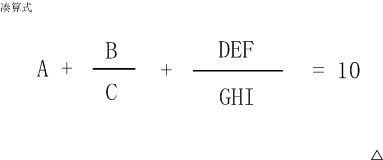6+8/3+952/714 就是一种解法，
5+3/1+972/486 是另一种解法。



### 思路

#include<iostream>
#include<vector>
using namespace std;
bool st;
vector<int> vec;
int ans;
double get(int l,int r)
{
int res = 0;
for(int i=l;i<=r;++i)
{
res = res*10+vec[i];
}
return res;
}
bool check()
{
double A = get(0,0);
double B = get(1,1);
double C = get(2,2);
double DEF = get(3,5);
double GHI = get(6,8);
if(A+B/C+DEF/GHI==10)
return true;
return false;
}
void dfs(int n)
{
if(n==9)
{
if(check())
ans++;
return;
}
for(int i=1;i<=9;++i)
{
if(!st[i])
{
vec.push_back(i);
st[i]=true;
dfs(n+1);
st[i]=false;
vec.pop_back();
}
}
}
int main()
{
dfs(0);
cout<<ans<<endl;
return 0;
}


29

## 4. 快速排序

### 答案

swap(a,j,p);

## 5.抽签

X星球要派出一个5人组成的观察团前往W星。

A国最多可以派出4人。
B国最多可以派出2人。
C国最多可以派出2人。
….

DEFFF
CEFFF
CDFFF
CDEFF
CCFFF
CCEFF
CCDFF
CCDEF
BEFFF
BDFFF
BDEFF
BCFFF
BCEFF
BCDFF
BCDEF
….
(以下省略，总共101行)

#include <stdio.h>
#define N 6
#define M 5
#define BUF 1024

void f(int a[], int k, int m, char b[])
{
int i,j;

if(k==N){
b[M] = 0;
if(m==0) printf("%s\n",b);
return;
}

for(i=0; i<=a[k]; i++){
for(j=0; j<i; j++) b[M-m+j] = k+'A';
______________________;  //填空位置
}
}
int main()
{
int  a[N] = {4,2,2,1,1,3};
char b[BUF];
f(a,0,M,b);
return 0;
}


### 答案

#include <stdio.h>
#define N 6
#define M 5
#define BUF 1024

void f(int a[], int k, int m, char b[])
{
int i,j;

if(k==N){
b[M] = 0;
if(m==0) printf("%s\n",b);
return;
}

for(i=0; i<=a[k]; i++){
for(j=0; j<i; j++) b[M-m+j] = k+'A';
f(a,k+1,m-j,b);  //填空位置
}
}
int main()
{
int  a[N] = {4,2,2,1,1,3};
char b[BUF];
f(a,0,M,b);
return 0;
}


## 6.方格填数

（左右、上下、对角都算相邻）一共有多少种可能的填数方案？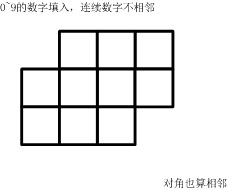### 代码

#include<iostream>
#include<algorithm>
using namespace std;
int a;
int b;
int dx[] = {-1,-1,0,1,1,1,0,-1};
int dy[] = {0,1,1,1,0,-1,-1,-1};
bool check()
{
//填入数字
int k=0;
for(int i=0;i<3;++i)
{
for(int j=0;j<4;++j)
{
if((i==0&&j==0)||(i==2&&j==3))continue;
b[i][j]=a[k++];
}
}
//开始判断
for(int i=0;i<3;++i)
{
for(int j=0;j<4;++j)
{
if((i==0&&j==0)||(i==2&&j==3))continue;//注意这里，这两个不需要判断
for(int d=0;d<8;++d)
{
int x = i+dx[d];
int y = j+dy[d];
if(x<0||x>=3||y<0||y>=4)continue;
if((x==0&&y==0)||(x==2&&y==3))continue;//谨记那两个空位也是不合法位置
if(b[x][y]==b[i][j]+1)return false;
}
}
}
return true;
}
int main()
{
int ans = 0;
for(int i=0;i<10;++i)a[i]=i;
do{
if(check())
ans++;
}while(next_permutation(a,a+10));
cout<<ans<<endl;
return 0;
}


1580

## 7.剪邮票

如【图1.jpg】, 有12张连在一起的12生肖的邮票。

（仅仅连接一个角不算相连）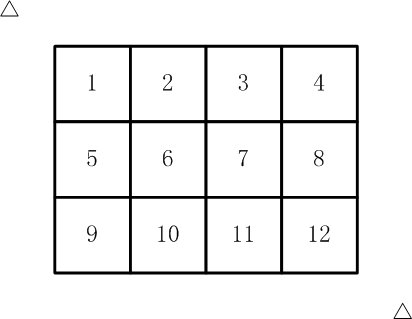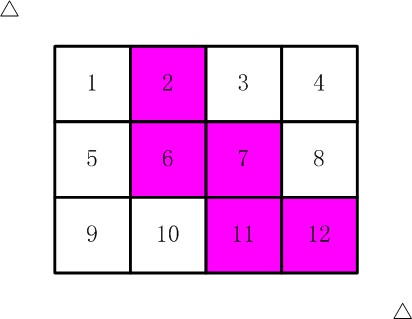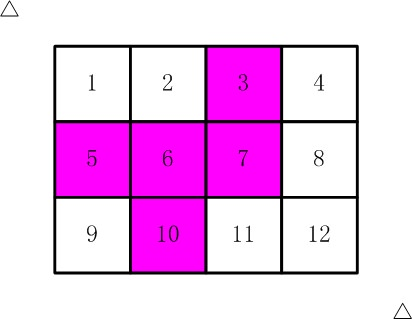### 代码

#include<iostream>
#include<algorithm>
#include<queue>
#include<cstring>
using namespace std;
#define x first
#define y second
typedef pair<int,int> PII;
int a={0,0,0,0,0,0,0,1,1,1,1,1};
int dx[] = {-1,0,1,0};
int dy[] = {0,1,0,-1};
bool st;
bool bfs()
{
queue<PII> q;
memset(st,0,sizeof st);
for(int i=0;i<3;++i)
{
for(int j=0;j<4;++j)
{
if(a[i][j]==1)
{
q.push(make_pair(i,j));
st[i][j]=true;
i=100;
break;
}
}
}
int res = 0;
while(q.size())
{
PII t = q.front();
q.pop();
res++;
for(int i=0;i<4;++i)
{
int aa = dx[i]+t.x;
int bb = dy[i]+t.y;
if(aa<0||aa>=3||bb<0||bb>=4)continue;
if(st[aa][bb])continue;
if(a[aa][bb]==0)continue;
q.push(make_pair(aa,bb));
st[aa][bb]=true;
}
}
return res==5;
}
int main()
{
int ans = 0;
do{
if(bfs())
ans++;
}while(next_permutation(a,a+12));
cout<<ans<<endl;
return 0;
}


116

## 8.四平方和定理

四平方和定理，又称为拉格朗日定理：

5 = 0^2 + 0^2 + 1^2 + 2^2
7 = 1^2 + 1^2 + 1^2 + 2^2
（^符号表示乘方的意思）

0 <= a <= b <= c <= d



### 代码

#include<iostream>
#include<algorithm>
using namespace std;
const int N = 2500010;
struct Node{
int l,r,w;
bool operator<(const Node& W)const
{
if(W.w!=w)return w<W.w;
if(W.l!=l)return l<W.l;
return r<W.r;
}
}sum[N];
int main()
{
int n;
cin>>n;
int k=0;
for(int c=0;c*c<=n;++c)
{
for(int d=c;c*c+d*d<=n;++d)
{
sum[k].w=c*c+d*d;
sum[k].l=c;
sum[k].r=d;
k++;
}
}
sort(sum,sum+k);
for(int a=0;a*a<=n;++a)
{
for(int b=a;b*b+a*a<=n;++b)
{
int t = n-a*a-b*b;
int l=0,r=k-1;
while(l<r)
{
int mid = l+r >>1;
if(sum[mid].w<t)l=mid+1;
else r=mid;
}
if(sum[l].w==t)
{
cout<<a<<" "<<b<<" "<<sum[l].l<<" "<<sum[l].r<<endl;
return 0;
}
}
}
return 0;
}


## 9.交换瓶子

有N个瓶子，编号 1 ~ N，放在架子上。

2 1 3 5 4

1 2 3 4 5



### 代码

#include<iostream>
using namespace std;
const int N = 10010;
int a[N];
int n;
int main()
{
cin>>n;
for(int i=1;i<=n;++i)cin>>a[i];
int ans = 0;
for(int i=1;i<=n;++i)
{
while(a[i]!=i)
{
swap(a[a[i]],a[i]);
ans++;
}
}
cout<<ans<<endl;
return 0;
}


## 10.最大比例

X星球的某个大奖赛设了M级奖励。每个级别的奖金是一个正整数。

16,24,36,54



### 代码

#include<iostream>
#include<algorithm>
using namespace std;
const int N = 110;
typedef long long LL;
LL up[N],down[N];
LL a[N];
LL gcd(LL a,LL b)
{
return b?gcd(b,a%b):a;
}
LL qgcd(LL a,LL b)
{
if(a<b)swap(a,b);
if(b==1)return a;
return qgcd(b,a/b);
}
int n;
int len;
int main()
{
cin>>n;
for(int i=0;i<n;++i)cin>>a[i];
sort(a,a+n);
for(int i=1;i<n;++i)
{
LL d = gcd(a[i],a[i-1]);
up[len]=a[i]/d;
down[len]=a[i-1]/d;
len++;
}
LL u = 1,d=1;
for(int i=0;i<len;++i)
{
u = qgcd(u,up[i]);
d = qgcd(d,down[i]);
}
cout<<u<<"/"<<d<<endl;
return 0;
}


mlxg
5天前

# 第八届蓝桥杯C++B组省赛题

## 1.购物单

### 代码

#include<iostream>
#include<fstream>
using namespace std;

int main()
{
ifstream fin("test01.txt");
double price,count;
double money = 0;
while(fin>>price>>count)
{
if(count<10)count*=10;
money+=price*count*0.01;
}
cout<<money<<endl;
int ans = 0;
while(ans<money)ans+=100;
printf("%d",ans);
return 0;
}


5200

## 2. 等差素数列

2,3,5,7,11,13,....是素数序列。 类似：7,37,67,97,127,157 这样完全由素数组成的等差数列，叫等差素数数列。 上边的数列公差为30，长度为6。 2004年，格林与华人陶哲轩合作证明了：存在任意长度的素数等差数列。 这是数论领域一项惊人的成果！ 有这一理论为基础，请你借助手中的计算机，满怀信心地搜索： 长度为10的等差素数列，其公差最小值是多少？ 注意：需要提交的是一个整数，不要填写任何多余的内容和说明文字。

### 代码

#include<iostream>
using namespace std;
const int N = 100010;
bool st[N];
int prime[N],cnt;
void init()
{
for(int i=2;i<=N;++i)
{
if(!st[i])prime[cnt++]=i;
for(int j=0;j<cnt&&prime[j]*i<=N;++j)
{
st[i*prime[j]]=true;
if(i%prime[j]==0)
break;
}
}
}
bool isok(int a,int d)
{
for(int i=0;i<10;++i)
{
if(a+d*i>N||st[a+d*i])
{
return false;
}
}
return true;
}
int main()
{
init();
for(int k=1;;k++)
{
for(int i=2;i<=N;++i)
{
if(!st[i]&&isok(i,k))
{
cout<<k<<endl;
return 0;
}
}
}
return 0;
}


210

## 3.承压计算

X星球的高科技实验室中整齐地堆放着某批珍贵金属原料。

                             7
5 8
7 8 8
9 2 7 2
8 1 4 9 1
8 1 8 8 4 1
7 9 6 1 4 5 4
5 6 5 5 6 9 5 6
5 5 4 7 9 3 5 5 1
7 5 7 9 7 4 7 3 3 1
4 6 4 5 5 8 8 3 2 4 3
1 1 3 3 1 6 6 5 5 4 4 2
9 9 9 2 1 9 1 9 2 9 5 7 9
4 3 3 7 7 9 3 6 1 3 8 8 3 7
3 6 8 1 5 3 9 5 8 3 8 1 8 3 3
8 3 2 3 3 5 5 8 5 4 2 8 6 7 6 9
8 1 8 1 8 4 6 2 2 1 7 9 4 2 3 3 4
2 8 4 2 2 9 9 2 8 3 4 9 6 3 9 4 6 9
7 9 7 4 9 7 6 6 2 8 9 4 1 8 1 7 2 1 6
9 2 8 6 4 2 7 9 5 4 1 2 5 1 7 3 9 8 3 3
5 2 1 6 7 9 3 2 8 9 5 5 6 6 6 2 1 8 7 9 9
6 7 1 8 8 7 5 3 6 5 4 7 3 4 6 7 8 1 3 2 7 4
2 2 6 3 5 3 4 9 2 4 5 7 6 6 3 2 7 2 4 8 5 5 4
7 4 4 5 8 3 3 8 1 8 6 3 2 1 6 2 6 4 6 3 8 2 9 6
1 2 4 1 3 3 5 3 4 9 6 3 8 6 5 9 1 5 3 2 6 8 8 5 3
2 2 7 9 3 3 2 8 6 9 8 4 4 9 5 8 2 6 3 4 8 4 9 3 8 8
7 7 7 9 7 5 2 7 9 2 5 1 9 2 6 5 3 9 3 5 7 3 5 4 2 8 9
7 7 6 6 8 7 5 5 8 2 4 7 7 4 7 2 6 9 2 1 8 2 9 8 5 7 3 6
5 9 4 5 5 7 5 5 6 3 5 3 9 5 8 9 5 4 1 2 6 1 4 3 5 3 2 4 1
X X X X X X X X X X X X X X X X X X X X X X X X X X X X X X


### 代码

#include<iostream>
#include<fstream>
using namespace std;

const int N = 35;
double f[N][N];
int main()
{
ifstream fin("test03.txt");
for(int i=1;i<=29;++i)
{
for(int j=1;j<=i;++j)
{
fin>>f[i][j];
}
}
for(int i=2;i<=30;++i)
{
for(int j=1;j<=i;++j)
{
f[i][j]+=f[i-1][j-1]/2+f[i-1][j]/2;
}
}
double maxv = -1e9,minv = 1e9;
for(int i=1;i<=30;++i)
{
if(f[i]>maxv)maxv = f[i];
if(f[i]<minv)minv = f[i];
}
printf("%.0f",2086458231/minv*maxv);
return 0;
}


72665192664

## 4.方格分割

6x6的方格，沿着格子的边线剪开成两部分。

### 代码

#include<iostream>
using namespace std;
const int N = 7;
bool st[N][N];
int dx[]={-1,0,1,0};
int dy[]={0,1,0,-1};
int ans;
void dfs(int x,int y)
{
if(x==0||x==6||y==0||y==6)//到达了边界
{
ans++;
return;
}
for(int i=0;i<4;++i)
{
int a = dx[i]+x;
int b = dy[i]+y;
if(st[a][b])continue;//不能走走过的地方
st[a][b]=true;
st[6-a][6-b]=true;//防止交叉，两个都要标记
dfs(a,b);
st[a][b]=false;
st[6-a][6-b]=false;
}
}
int main()
{
st=true;
dfs(3,3);
cout<<ans/4<<endl;
return 0;
}


509

## 7.日期问题

小明正在整理一批历史文献。这些历史文献中出现了很多日期。小明知道这些日期都在1960年1月1日至2059年12月31日。令小明头疼的是，这些日期采用的格式非常不统一，有采用年/月/日的，有采用月/日/年的，还有采用日/月/年的。更加麻烦的是，年份也都省略了前两位，使得文献上的一个日期，存在很多可能的日期与其对应。

----

----

----
02/03/04

----
2002-03-04
2004-02-03
2004-03-02

CPU消耗  < 1000ms

main函数需要返回0;



### 代码

#include<iostream>
using namespace std;
int days[]={0,31,28,31,30,31,30,31,31,30,31,30,31};
int first,second,third;
bool isleap(int year)
{
if((year%4==0&&year%100!=0)||(year%400==0))
return true;
return false;
}
int modify(int x)
{
int year = x/10000;
int month = x%10000/100;
int day = x%100;
day++;
int t = days[month];
if(month==2&&isleap(year))
t+=1;
if(day>t)
{
day=1;
month++;
}
if(month>12)
{
month=1;
year++;
}
return year*10000+month*100+day;
}
bool check(int x)
{
int year = x/10000%100;
int month = x%10000/100;
int day = x%100;
if((first==year&&second==month&&third==day)||(first==month&&second==day&&third==year)||(first==day&&second==month&&third==year))
return true;
return false;
}
int main()
{
scanf("%d/%d/%d",&first,&second,&third);
int start = 19600101,end = 20591231;
for(int i=start;i<=end;i=modify(i))
{
if(check(i))
printf("%d-%02d-%02d\n",i/10000,i%10000/100,i%100);
}
}


## 8.包子凑数

小明几乎每天早晨都会在一家包子铺吃早餐。他发现这家包子铺有N种蒸笼，其中第i种蒸笼恰好能放Ai个包子。每种蒸笼都有非常多笼，可以认为是无限笼。

----

----

2
4
5

6

2
4
6

INF

CPU消耗  < 1000ms

main函数需要返回0;



### 代码

#include<iostream>
using namespace std;
const int N = 110,M = N*N;
int n;
int v[N];
int f[M];
int gcd(int a,int b)
{
return b?gcd(b,a%b):a;
}
int main()
{
cin>>n;
for(int i=1;i<=n;++i)cin>>v[i];
int d = 0;
for(int i=1;i<=n;++i)d=gcd(d,v[i]);
if(d>1)
{
puts("INF");
}
else{
f=true;
for(int i=1;i<=n;++i)
{
for(int j=v[i];j<M;++j)
{
f[j]|=f[j-v[i]];
}
}
int ans = 0;
for(int i=0;i<M;++i)
{
if(!f[i])ans++;
}
cout<<ans<<endl;
}
return 0;
}


## 9.分巧克力

标题： 分巧克力

儿童节那天有K位小朋友到小明家做客。小明拿出了珍藏的巧克力招待小朋友们。
小明一共有N块巧克力，其中第i块是Hi x Wi的方格组成的长方形。

为了公平起见，小明需要从这 N 块巧克力中切出K块巧克力分给小朋友们。切出的巧克力需要满足：

1. 形状是正方形，边长是整数
2. 大小相同

2 10
6 5
5 6

2

CPU消耗  < 1000ms

main函数需要返回0;



### 代码

#include<iostream>
using namespace std;
const int N = 100010;
int w[N],h[N];
int n,k;
bool check(int x)
{
long long res = 0;
for(int i=1;i<=n;++i)
{
res+=(w[i]/x)*(h[i]/x);
}
return res>=k;
}
int main()
{
cin>>n>>k;
for(int i=1;i<=n;++i)cin>>w[i]>>h[i];
int l=1,r=N;
while(l<r)
{
int mid = l+r+1 >>1;
if(check(mid))l=mid;
else r=mid-1;
}
cout<<l<<endl;
return 0;
}


## 10.k倍区间

给定一个长度为N的数列，A1, A2, ... AN，如果其中一段连续的子序列Ai, Ai+1, ... Aj(i <= j)之和是K的倍数，我们就称这个区间[i, j]是K倍区间。

-----

-----

5 2
1
2
3
4
5

6

CPU消耗  < 2000ms

main函数需要返回0;



### 代码

#include<iostream>
using namespace std;
const int N = 100010;
int n,k;
long long a[N],sum[N];
int main()
{
cin>>n>>k;
for(int i=1;i<=n;++i)cin>>a[i];
sum=1;
long long res = 0;
for(int i=1;i<=n;++i)
{
a[i]+=a[i-1];
res+=sum[a[i]%k]++;
}
cout<<res<<endl;
return 0;
}


mlxg
7天前

# 第九届蓝桥杯题解（C++B组）

## 1.第几天

2000年的1月1日，是那一年的第1天。

### 代码

#include<iostream>
using namespace std;
int days[] = {31,28,31,30,31,30,31,31,30,31,30,31};
bool isleap(int year)
{
if((year%4==0&&year%100!=0)||year%400==0)
return true;
return false;
}
int main()
{
int year,month,day;
int ans = 0;
cin>>year>>month>>day;
for(int i=0;i<month-1;++i)//下标是从0开始的
{
ans += days[i];
if(i==1&&isleap(year))
ans+=1;
}
cout<<ans+day<<endl;
return 0;
}


125

## 2.明码

16点阵的字库把每个汉字看成是16x16个像素信息。并把这些信息记录在字节中。

第1字节，第2字节

....



4 0 4 0 4 0 4 32 -1 -16 4 32 4 32 4 32 4 32 4 32 8 32 8 32 16 34 16 34 32 30 -64 0
16 64 16 64 34 68 127 126 66 -124 67 4 66 4 66 -124 126 100 66 36 66 4 66 4 66 4 126 4 66 40 0 16
4 0 4 0 4 0 4 32 -1 -16 4 32 4 32 4 32 4 32 4 32 8 32 8 32 16 34 16 34 32 30 -64 0
0 -128 64 -128 48 -128 17 8 1 -4 2 8 8 80 16 64 32 64 -32 64 32 -96 32 -96 33 16 34 8 36 14 40 4
4 0 3 0 1 0 0 4 -1 -2 4 0 4 16 7 -8 4 16 4 16 4 16 8 16 8 16 16 16 32 -96 64 64
16 64 20 72 62 -4 73 32 5 16 1 0 63 -8 1 0 -1 -2 0 64 0 80 63 -8 8 64 4 64 1 64 0 -128
0 16 63 -8 1 0 1 0 1 0 1 4 -1 -2 1 0 1 0 1 0 1 0 1 0 1 0 1 0 5 0 2 0
2 0 2 0 7 -16 8 32 24 64 37 -128 2 -128 12 -128 113 -4 2 8 12 16 18 32 33 -64 1 0 14 0 112 0
1 0 1 0 1 0 9 32 9 16 17 12 17 4 33 16 65 16 1 32 1 64 0 -128 1 0 2 0 12 0 112 0
0 0 0 0 7 -16 24 24 48 12 56 12 0 56 0 -32 0 -64 0 -128 0 0 0 0 1 -128 3 -64 1 -128 0 0

### 代码

#include<iostream>
#include<bitset>
using namespace std;

int main()
{
for(int k=0;k<10;++k)//k个汉字
{
for(int i=0;i<16;++i)//每次输入两个字节，供需输入16次
{
int num1,num2;
cin>>num1>>num2;
bitset<8> bit1(num1),bit2(num2);
string str1 = bit1.to_string();
string str2 = bit2.to_string();
for(int i=0;i<8;++i)
if(str1[i]=='0')
cout<<" ";
else
cout<<"*";
for(int i=0;i<8;++i)
if(str2[i]=='0')
cout<<" ";
else
cout<<"*";
cout<<endl;//换个行
}
}
return 0;
}


387 420 489

## 3.乘积尾零

5650 4542 3554 473 946 4114 3871 9073 90 4329
2758 7949 6113 5659 5245 7432 3051 4434 6704 3594
9937 1173 6866 3397 4759 7557 3070 2287 1453 9899
1486 5722 3135 1170 4014 5510 5120 729 2880 9019
2049 698 4582 4346 4427 646 9742 7340 1230 7683
5693 7015 6887 7381 4172 4341 2909 2027 7355 5649
6701 6645 1671 5978 2704 9926 295 3125 3878 6785
2066 4247 4800 1578 6652 4616 1113 6205 3264 2915
3966 5291 2904 1285 2193 1428 2265 8730 9436 7074
689 5510 8243 6114 337 4096 8199 7313 3685 211

### 代码

#include<iostream>
#include<bitset>
using namespace std;

int main()
{
int two=0,five=0;
for(int i=0;i<100;++i)
{
int num;
cin>>num;
while(num%2==0)
{
two++;
num/=2;
}
while(num%5==0)
{
five++;
num/=5;
}
}
cout<<min(two,five)<<endl;
return 0;
}


31

## 4.测试次数

x星球的居民脾气不太好，但好在他们生气的时候唯一的异常举动是：摔手机。

x星球有很多高耸入云的高塔，刚好可以用来做耐摔测试。塔的每一层高度都是一样的，与地球上稍有不同的是，他们的第一层不是地面，而是相当于我们的2楼。

### 思路

dp[i][j]包括哪几种情况呢？第i部手机在j个层楼扔下去的情况之和。若是在第k层(这个第k并不是整体上的第k)扔下去，那么为摔坏和没摔坏，分别是dp[i-1][k-1]和dp[i][j-k]，由于我们运气背，所以我们总是会需要max(dp[i-1][k-1],dp[i][j-k]).

### 代码

#include<iostream>
#include<bitset>
using namespace std;
const int N = 10 ,M = 1010;
int f[N][M];
int main()
{
int n,m;
cin>>n>>m;
for(int i=1;i<=n;++i)
{
for(int j=1;j<=m;++j)
{
f[i][j]=j;
}
}
for(int i=2;i<=n;++i)
{
for(int j=1;j<=m;++j)
{
for(int k=1;k<=j;++k)
{
f[i][j]=min(f[i][j],max(f[i-1][k-1],f[i][j-k])+1);
}
}
}
cout<<f[n][m]<<endl;
return 0;
}


19

## 5.快速排序

#include <stdio.h>

int quick_select(int a[], int l, int r, int k) {
int p = rand() % (r - l + 1) + l;
int x = a[p];
{int t = a[p]; a[p] = a[r]; a[r] = t;}
int i = l, j = r;
while(i < j) {
while(i < j && a[i] < x) i++;
if(i < j) {
a[j] = a[i];
j--;
}
while(i < j && a[j] > x) j--;
if(i < j) {
a[i] = a[j];
i++;
}
}
a[i] = x;
p = i;
if(i - l + 1 == k) return a[i];
if(i - l + 1 < k) return quick_select( _____________________________ ); //填空
else return quick_select(a, l, i - 1, k);
}

int main()
{
int a[] = {1, 4, 2, 8, 5, 7, 23, 58, 16, 27, 55, 13, 26, 24, 12};
printf("%d\n", quick_select(a, 0, 14, 5));
return 0;
}


### 答案

a,i+1,r,k-(i-l+1)

## 6.递增三元组

A = [A1, A2, … AN],
B = [B1, B2, … BN],
C = [C1, C2, … CN]，

1 <= i, j, k <= N

Ai < Bj < Ck

【输入格式】

【输出格式】

【样例输入】
3
1 1 1
2 2 2
3 3 3

【样例输出】
27

### 代码

#include<iostream>
#include<algorithm>
using namespace std;
const int N = 100010;
int a[N];
int b[N];
int c[N];
int n;
int main()
{
cin>>n;
for(int i=0;i<n;++i)cin>>a[i];
for(int i=0;i<n;++i)cin>>b[i];
for(int i=0;i<n;++i)cin>>c[i];
sort(a,a+n);
sort(c,c+n);
long long res = 0;
for(int i=0;i<n;++i)
{
long long t1 = lower_bound(a,a+n,b[i])-a;
long long t2 = n-(upper_bound(c,c+n,b[i])-c);
res+=t1*t2;
}
cout<<res<<endl;
return 0;
}


## 7.螺旋折线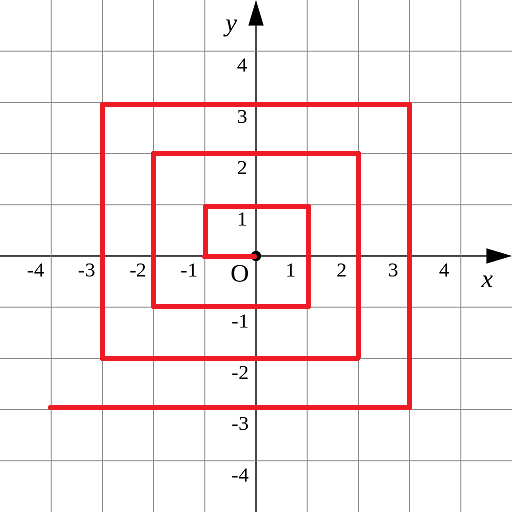【输入格式】
X和Y

【输出格式】

【样例输入】
0 1

【样例输出】
3

### 代码

#include<iostream>
#include<algorithm>
using namespace std;
typedef long long LL;
int main()
{
LL x,y;
cin>>x>>y;
LL len = max(abs(x),abs(y));
LL res = (len-1)*len*4;
if(x==-len)res+=(y+len);
else if(y==len)res+=2*len+x+len;
else if(x==len)res+=4*len+len-y;
else res+=6*len+len-x;
cout<<res<<endl;
return 0;
}


## 8.日志统计

ts id

1≤K≤N≤105,
0≤ts,id≤105,
1≤D≤10000

7 10 2
0 1
0 10
10 10
10 1
9 1
100 3
100 3

1
3

### 代码

#include<iostream>
#include<algorithm>
using namespace std;
const int N = 100010;
#define x first
#define y second
typedef pair<int,int> PII;
PII query[N];
int cnt[N];//统计时间区间内的点赞量
bool st[N];
int n,d,k;
int main()
{
cin>>n>>d>>k;
for(int i=0;i<n;++i)
{
int a,b;
cin>>a>>b;
query[i]=make_pair(a,b);
}
sort(query,query+n);
for(int i=0,j=0;i<n;++i)
{
cnt[query[i].y]++;
while(query[i].x-query[j].x>=d)
{
cnt[query[j].y]--;
j++;
}
if(cnt[query[i].y]>=k)st[query[i].y]=true;
}
for(int i=0;i<N;++i)
if(st[i])
cout<<i<<endl;
return 0;
}


## 9.全球变暖

.......
.##....
.##....
....##.
..####.
…###.
.......

.......
.......
.......
.......
....#..
.......
.......

【输入格式】

【输出格式】

### 代码

#include<iostream>
#include<algorithm>
#include<queue>
using namespace std;
const int N = 1010;
typedef pair<int,int> PII;
#define x first
#define y second
char g[N][N];
bool st[N][N];
int dx[] = {-1,0,1,0};
int dy[] = {0,1,0,-1};
int n;
bool bfs(int sx,int sy)
{
queue<PII> q;
int res = 0;//记录存活的小方块
q.push(make_pair(sx,sy));
st[sx][sy]=true;
while(q.size())
{
PII t = q.front();
q.pop();
bool flag = true;
for(int i=0;i<4;++i)
{
int a = t.x+dx[i];
int b = t.y+dy[i];
if(a<0||a>=n||b<0||b>=n)continue;
if(st[a][b])
continue;
if(g[a][b]=='.')
{
flag = false;
continue;
}
q.push({a,b});
st[a][b]=true;
}
if(flag)
res++;
}
if(res>0) return true;
return false;
}
int main()
{
cin>>n;
int tol = 0,up = 0;//tol记录总的岛屿，up记录活着的岛屿
for(int i=0;i<n;++i)cin>>g[i];
for(int i=0;i<n;++i)
{
for(int j=0;j<n;++j)
{
if(!st[i][j]&&g[i][j]=='#')
{
tol++;
if(bfs(i,j))
up++;
}
}
}
cout<<tol-up<<endl;
return 0;
}


## 10.最大乘积

1≤K≤N≤105,
−105≤Ai≤105

5 3
-100000
-10000
2
100000
10000

999100009

5 3
-100000
-100000
-2
-100000
-100000

-999999829

### 代码

#include<iostream>
#include<algorithm>
#include<queue>
using namespace std;
const int N = 100010,mod = 1e9+9;
typedef long long LL;
LL a[N];
int n,k;
int main()
{
cin>>n>>k;
for(int i=0;i<n;++i)cin>>a[i];
sort(a,a+n);
LL res= 1;
int l=0,r=n-1;
int sign=1;
if(k%2)
{
res=a[r];
r--;
k--;
if(res<0)
sign=-1;//求最小的非负数
}
while(k)
{
LL x = a[l]*a[l+1],y = a[r]*a[r-1];
if(sign*x>sign*y)
{
res=x%mod*res%mod;
l+=2;
}
else
{
res=y%mod*res%mod;
r-=2;
}
k-=2;
}
cout<<res<<endl;
return 0;
}


mlxg
3个月前

### 算法思路

##### 代码
#include<iostream>
using namespace std;
const int N = 10010;
int a[N];
int n;
int main()
{
cin>>n;
for(int i=1;i<=n;++i)cin>>a[i];
int res = 0;
for(int i=1;i<=n;++i)
while(a[i]!=i)swap(a[a[i]],a[i]),res++;
cout<<res<<endl;
return 0;
}


mlxg
4个月前

#### 闫氏dp分析#include<iostream>
using namespace std;
const int N = 110,M=N*N;
bool f[M];
int gcd(int a,int b)
{
return b?gcd(b,a%b):a;
}
int v[N];
int n;
int main()
{
cin>>n;
for(int i=1;i<=n;++i)cin>>v[i];
int d=0;
for(int i=1;i<=n;++i)
{
d = gcd(d,v[i]);
}
if(d>1){
puts("INF");
return 0;
}
f=true;
for(int i=1;i<=n;++i)
{
for(int j=v[i];j<M;++j)
{
f[j]|=f[j-v[i]];
}
}
int res = 0;
for(int i=1;i<M;++i)
{
if(!f[i])
res++;
}
cout<<res<<endl;
return 0;
}


mlxg
4个月前

### 巧妙的矩阵构造

Fn= [fn,fn+1,sn]
Fn+1= [fn+1,fn+2,sn+1]
Fn*A = Fn+1

1 1 1
0 0 1
Fn = F1 * $A^{n-1}$

F1 = 1 1 1
0 0 0
0 0 0

#include<iostream>
#include<cstring>
using namespace std;
typedef long long LL;
const int N = 3;
LL n,m;
void mul(LL c[][N],LL a[][N],LL b[][N])//多维数组除了第一维 其他都要指定长度
{
LL temp[N][N]={0};
for(int i=0;i<N;++i)
{
for(int j=0;j<N;++j)
{
for(int k=0;k<N;++k)
{
temp[i][j]=(temp[i][j]+a[i][k]*b[k][j])%m;
}
}
}
memcpy(c,temp,sizeof temp);//形参sizeof 是不可行的
}
int main()
{
cin>>n>>m;
LL f[N][N]={1,1,1};
LL a[N][N]={
{0,1,0},
{1,1,1},
{0,0,1}
};
n--;
while(n)
{
if(n&1)mul(f,f,a);
mul(a,a,a);
n>>=1;
}
cout<<f<<endl;
return 0;
}


mlxg
4个月前

### 树形dp

f[u]=w[u]+max(子树的情况，0)

#include<iostream>
#include<cstring>
using namespace std;
typedef long long LL;
const int N = 100010,M=N*2;
int h[N],e[M],ne[M],w[N],idx;
int n;
LL f[N];
{
e[idx]=b;ne[idx]=h[a];h[a]=idx++;
}
void dfs(int u,int father)
{
f[u]=w[u];
for(int i=h[u];~i;i=ne[i])
{
int j = e[i];
if(j!=father)
{
dfs(j,u);
f[u]+=max(0ll,f[j]);
}
}
}

int main()
{
cin>>n;
memset(h,-1,sizeof h);
for(int i=1;i<=n;++i)cin>>w[i];
for(int i=0;i<n-1;++i){
int a,b;
cin>>a>>b;
}
dfs(2,-1);
LL res = f;
for(int i=2;i<=n;++i)res = max(res,f[i]);
cout<<res<<endl;
return 0;
}


mlxg
4个月前

## 注意下图右下角应该是 f[i+1][j-1]+2(s[i]==s[j])

### 闫氏dp法#include<iostream>
#include<cstring>
using namespace std;
const int N = 1010;
int f[N][N];
char str[N];
int main()
{
cin>>str+1;
int n = strlen(str+1);
for(int len=1;len<=n;++len)
{
for(int i=1;i+len-1<=n;++i)
{
int j = i+len-1;
if(len==1){f[i][j]=1;continue;}
f[i][j]=max(f[i+1][j],f[i][j-1]);
if(str[i]==str[j])
f[i][j]=max(f[i+1][j-1]+2,f[i][j]);
}
}
cout<<n-f[n]<<endl;
return 0;
}


mlxg
4个月前

### 闫氏dp分析法### 代码

#include<cstring>
#include<iostream>
using namespace std;

const int N = 110;
int f[N][N];
int n,k;
int main()
{
cin>>n>>k;
memset(f,-0x3f,sizeof f);
f=0;
for(int i=1;i<=n;++i)
{
int w;
cin>>w;
for(int j=0;j<k;++j)
{
f[i][j]=max(f[i-1][j],f[i-1][((j-w)%k+k)%k]+w);
}
}
cout<<f[n]<<endl;
return 0;
}